# Chapter 7 Chemical Reactions 7 1 Describing Reactions

• Slides: 66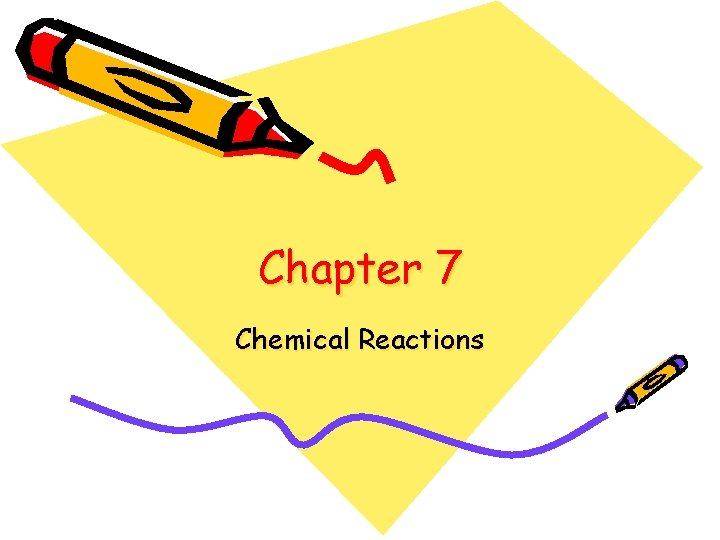Chapter 7 Chemical Reactions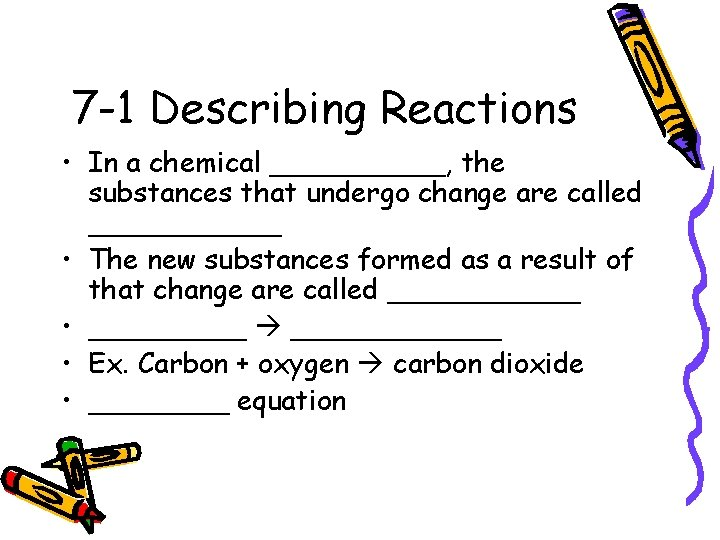7 -1 Describing Reactions • In a chemical _____, the substances that undergo change are called ______ • The new substances formed as a result of that change are called ______ • ______ • Ex. Carbon + oxygen carbon dioxide • ____ equation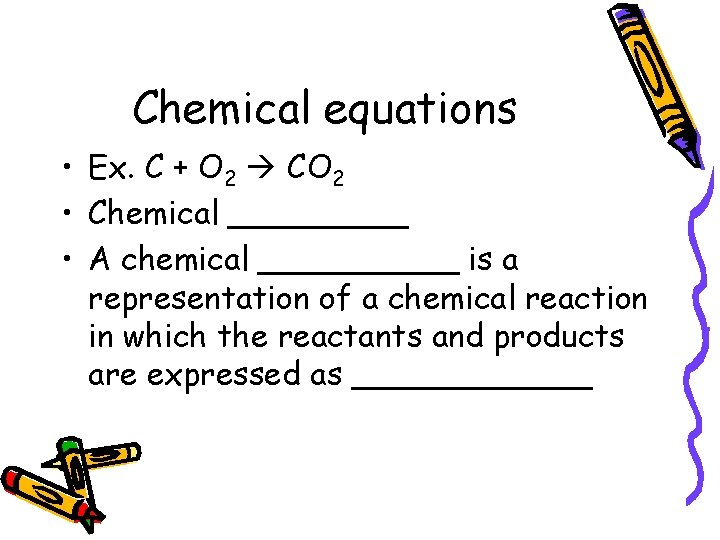Chemical equations • Ex. C + O 2 CO 2 • Chemical _____ • A chemical _____ is a representation of a chemical reaction in which the reactants and products are expressed as ______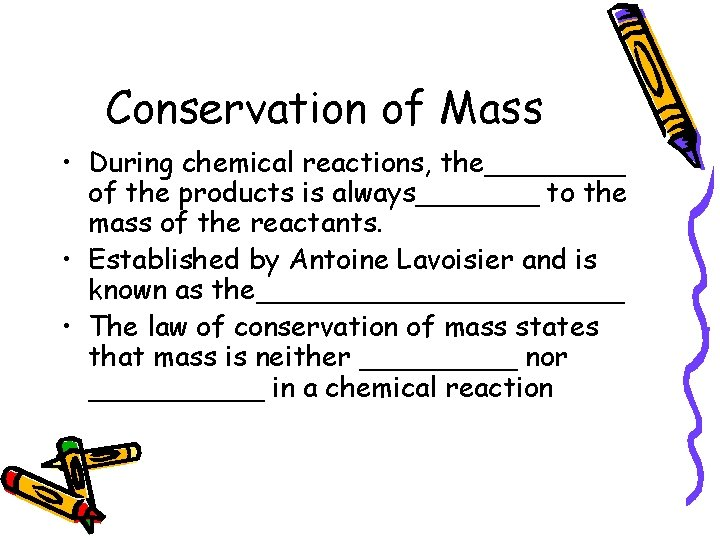Conservation of Mass • During chemical reactions, the____ of the products is always_______ to the mass of the reactants. • Established by Antoine Lavoisier and is known as the___________ • The law of conservation of mass states that mass is neither _____ nor _____ in a chemical reactionHow is mass conserved in chemical change? Activity • Follow the directions on page 191 in your book • Fill out following information • Mass of the bag and the water=______ • Mass of a square piece of paper with sides 10 cm_________ • Mass of the tablet and the paper together______ • Mass of just the tablet________ • The combined masses of the bag, the water, and the tablet______ • Mass of the bag and its contents_______Activity questions 1. How do you know whether a chemical change took place?Activity Question • What happened to the mass of the plastic bag and its contents after the bubbling stopped? • What might this information tell you about a chemical change?Balancing Equations 7 -1 Continued • In order to show that mass is conserved during a reaction, a chemical equation must be _____ • You can balance a chemical equation by changing the_____, the numbers that appear _____ the formulas • As you balance equations, you should never change the ______ in a formulaSteps to balancing equations 1. 2. 3. 4. 5. Count the _____ of atoms of each element on each side of the equation Ex: N 2 H 4 + O 2 N 2 + H 2 O Left side has ____ nitrogen, ____hydrogen, and ______ oxygen atoms The right side has ____nitrogen, _____hydrogen, and____oxygen atom The hydrogen and oxygen atoms need to be_______.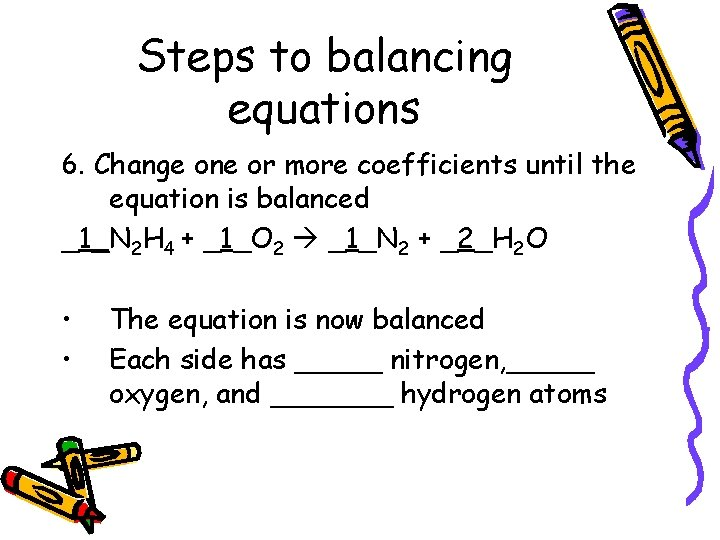Steps to balancing equations 6. Change one or more coefficients until the equation is balanced _1_N 2 H 4 + _1_O 2 _1_N 2 + _2_H 2 O • • The equation is now balanced Each side has _____ nitrogen, _____ oxygen, and _______ hydrogen atomsPractice Balancing equations problems 1. ___Na + ___H 2 O ____Na. OH + ___H 2 2. ___HCl + ___Ca. CO 3 ____Ca. Cl 2 +___CO 2 + ____H 2 O 3. ____Al + ____Cl 2 _____Al. Cl 3 4. ___Cu + _____O 2 _____Cu. O 5. ____H 2 O 2 ____H 2 O + ____O 2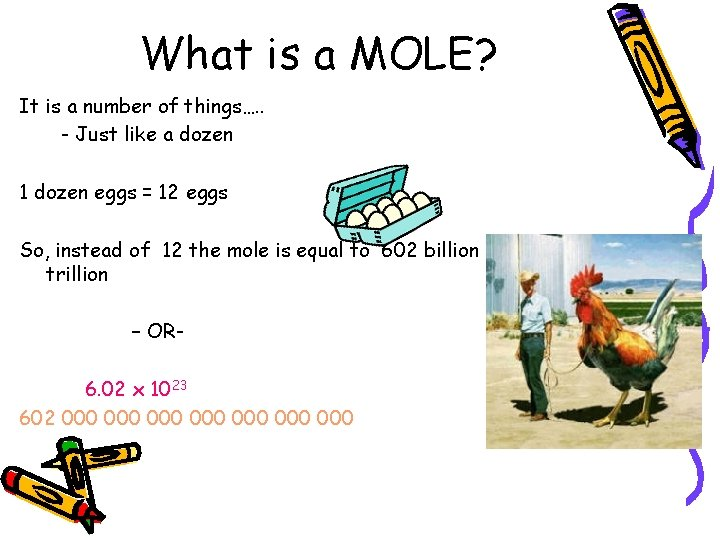What is a MOLE? It is a number of things…. . - Just like a dozen 1 dozen eggs = 12 eggs So, instead of 12 the mole is equal to 602 billion trillion – OR 6. 02 x 1023 602 000 000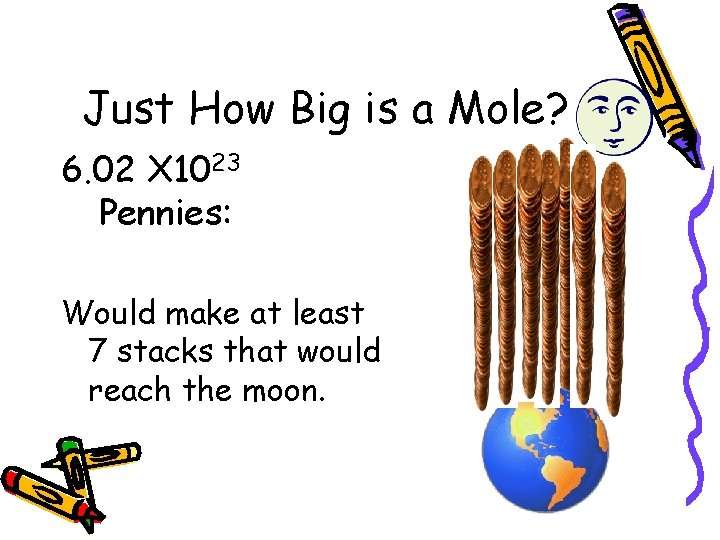Just How Big is a Mole? 6. 02 X 1023 Pennies: Would make at least 7 stacks that would reach the moon.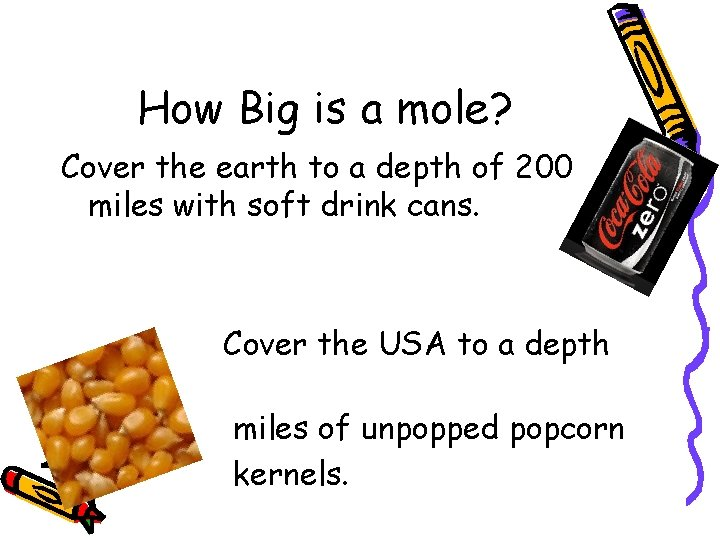How Big is a mole? Cover the earth to a depth of 200 miles with soft drink cans. of 9 Cover the USA to a depth miles of unpopped popcorn kernels.The mole is many things…. 1 dozen cookies = 12 cookies 1 mole of cookies = 6. 02 x 1023 cookies cars 1 dozen cars = 12 cars 1 mole of cars = 6. 02 x 10 23 1 dozen Al atoms = 12 Al atoms 1 mole of Al atoms = 6. 02 x 1023 atoms NOTE: the mole is abbreviated mol (gee, that’s a lot quicker to write……)Molar Mass • Mass of 1 ______ of a pure substance • Numerically equal to the atomic mass but expressed in _______ • The atomic mass of carbon is 12. 0____, so the molar mass of carbon is 12. 0_____• What is the molar mass of the following: • Potassium ? Nickel ?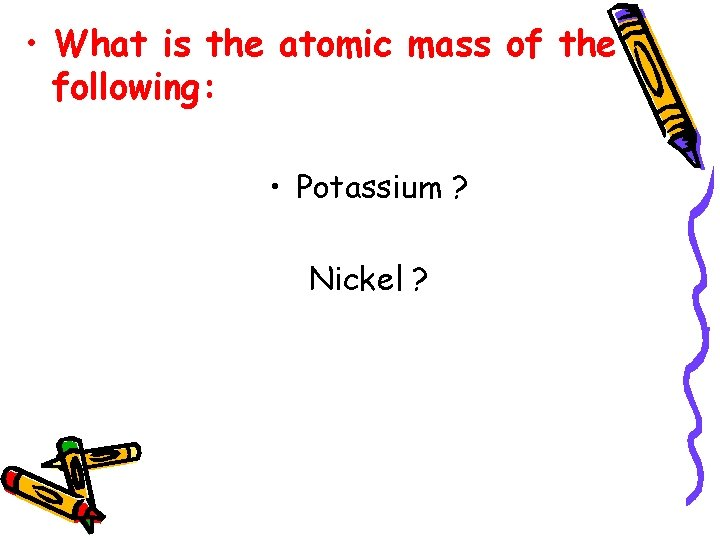• What is the atomic mass of the following: • Potassium ? Nickel ?Practice • 1. 2. 3. 4. What is the molar mass of the following: KCl C 2 H 6 Ca. CO 3 H 2 OHomework/More Practice • What is the molar mass of the following: 1. HCl 2. Na. Cl 3. Ca. O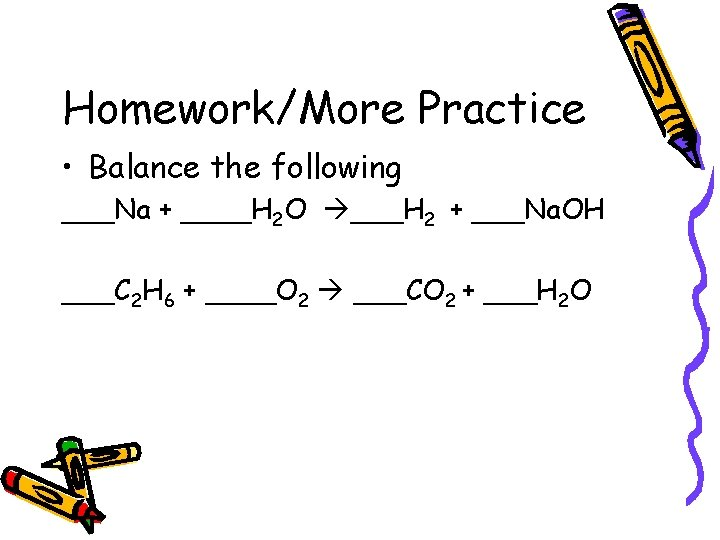Homework/More Practice • Balance the following ___Na + ____H 2 O ___H 2 + ___Na. OH ___C 2 H 6 + ____O 2 ___CO 2 + ___H 2 OBeaker Breaker • Calculate the molar mass of the following: • Mg. Cl 2 • Ca. Br 2Gram- Mole ConversionsHow many grams are needed to have 0. 852 moles gold? • 0. 852 mol Au 1 = ______g Au mol AuConvert 2. 50 moles of KCl. O 3 to grams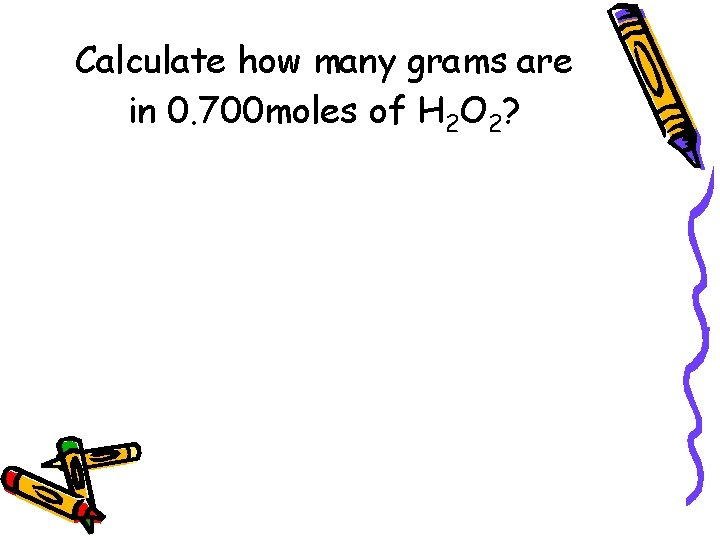Calculate how many grams are in 0. 700 moles of H 2 O 2?How many moles are in 55 g of lead? • g Pb 1 mol Pb g Pb • = _______moles PbHow many moles are in 86. 1 g sodium? • 86. 1 g ? mole Na = 1 ? g • • 86. 1 g mole Na = 1 g _______moles NaHow many moles are in 22 grams of copper metal?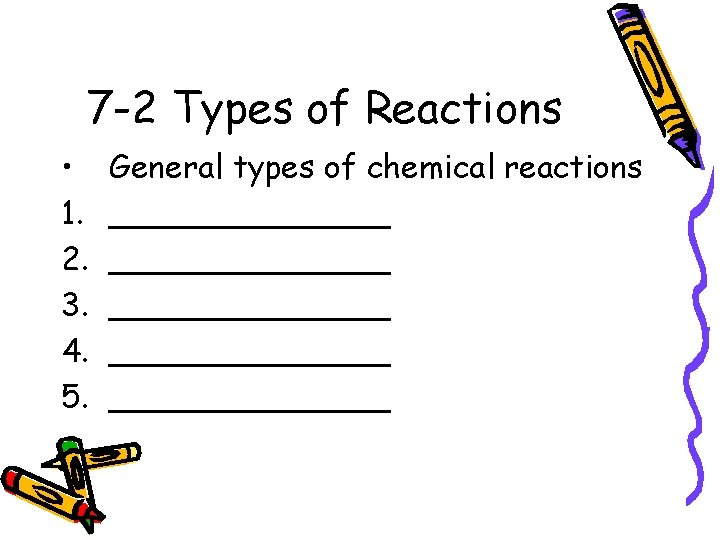7 -2 Types of Reactions • 1. 2. 3. 4. 5. General types of chemical reactions ______________ _______Single Replacement • ________ reaction is a reaction in which one element takes place of another element in a compound. • A + BC B + AC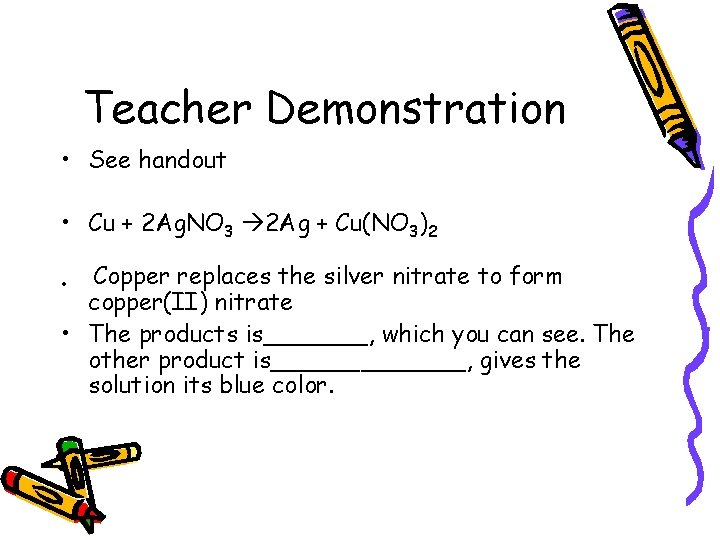Teacher Demonstration • See handout • Cu + 2 Ag. NO 3 2 Ag + Cu(NO 3)2 Copper replaces the silver nitrate to form copper(II) nitrate • The products is_______, which you can see. The other product is_______, gives the solution its blue color. •7 -2 Continued Reactions as Electron Transfer • The discovery of subatomic particles enabled scientists to classify certain chemical reactions as transfers of________ between atoms. • A reaction in which electrons are transferred from one reactant to another is called an __________ reaction, _____ reactionOxidation • Synthesis reactions, in which a______combines with_____, traditionally have been classified as oxidations. • 2 Ca +O 2 2_____ • Ca Ca+2 + 2 e • Calcium ____ two electrons • A reactant is_____ if it loses electronsReduction • As calcium atoms lose electrons during the synthesis of calcium oxide, the oxygen____ electrons • O + 2 e- O 2 • The process in which an element gains electrons during a chemical reaction is called______ • A reactant is said to be_______ if it gains electrons. • Oxidation and reduction always occur____.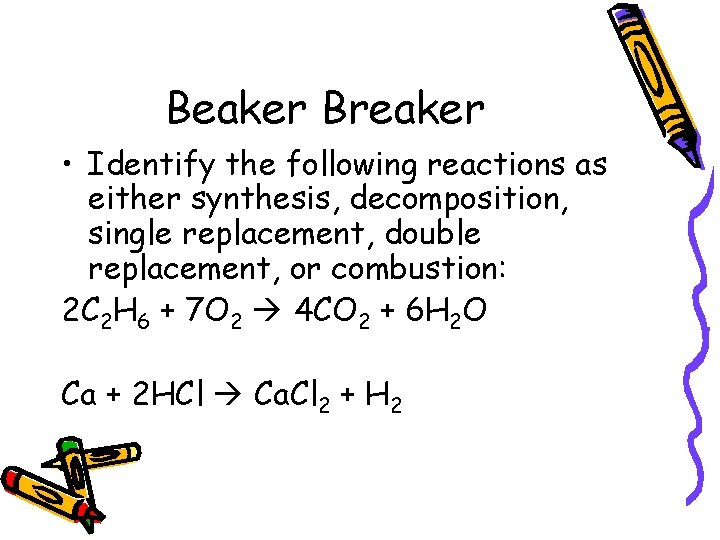Beaker Breaker • Identify the following reactions as either synthesis, decomposition, single replacement, double replacement, or combustion: 2 C 2 H 6 + 7 O 2 4 CO 2 + 6 H 2 O Ca + 2 HCl Ca. Cl 2 + H 2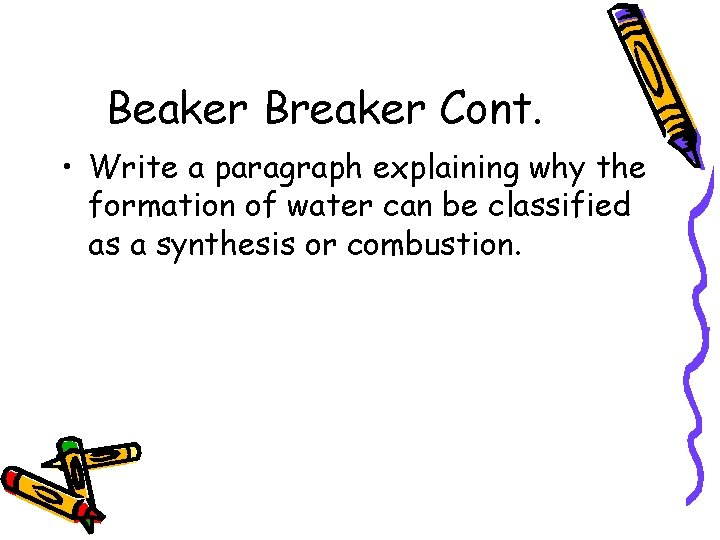Beaker Breaker Cont. • Write a paragraph explaining why the formation of water can be classified as a synthesis or combustion.7 -3 Energy Changes in Reactions Chemical Bonds and Energy • Heat produced by a_____(C 3 H 8) grill is a form of energy • Balance equation: • C 3 H 8 + ____O 2 ___CO 2 +___H 2 O • _____ will be added to the right side of the equation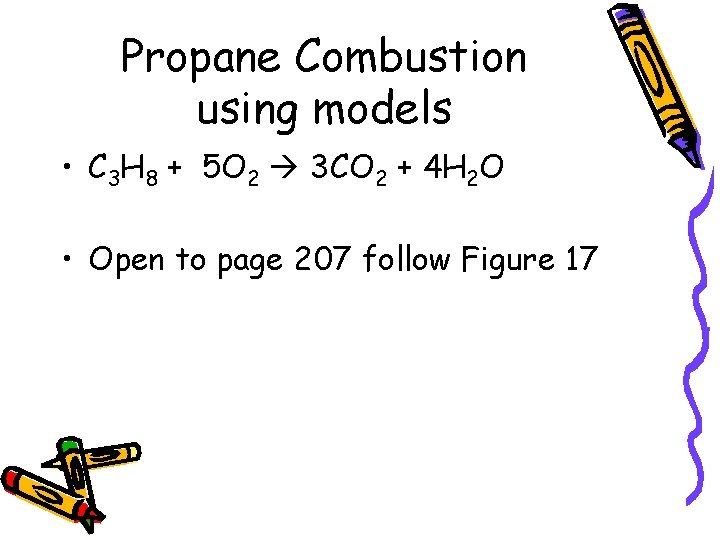Propane Combustion using models • C 3 H 8 + 5 O 2 3 CO 2 + 4 H 2 O • Open to page 207 follow Figure 17Chemical Energy • _______ is the energy stored in the chemical bonds of a substance. • Using the models to make C 3 H 8 (propane) • How many C-H bonds_____ • How many C-C bonds_____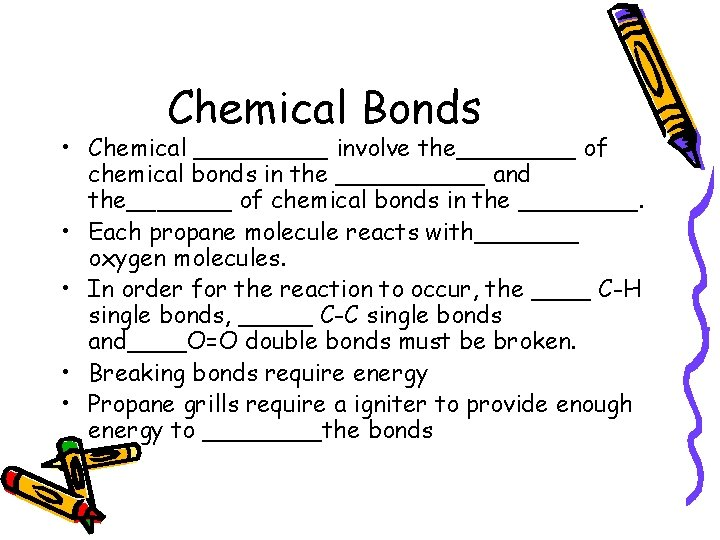Chemical Bonds • Chemical _____ involve the____ of chemical bonds in the _____ and the_______ of chemical bonds in the ____. • Each propane molecule reacts with_______ oxygen molecules. • In order for the reaction to occur, the ____ C-H single bonds, _____ C-C single bonds and____O=O double bonds must be broken. • Breaking bonds require energy • Propane grills require a igniter to provide enough energy to ____the bonds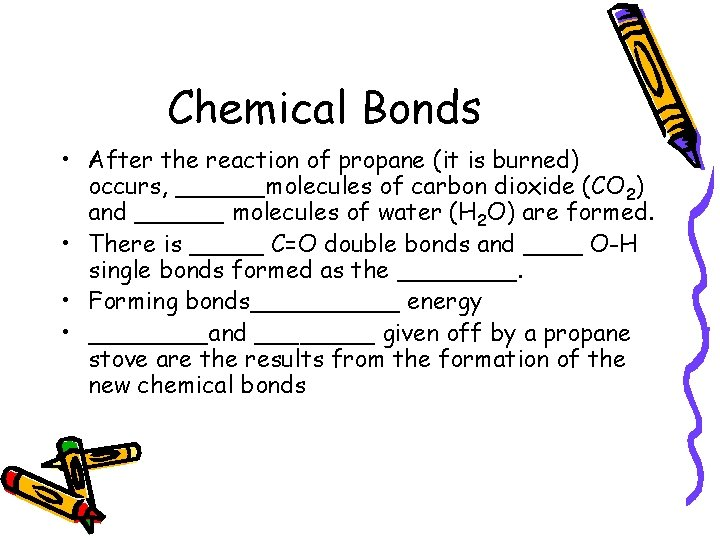Chemical Bonds • After the reaction of propane (it is burned) occurs, ______molecules of carbon dioxide (CO 2) and ______ molecules of water (H 2 O) are formed. • There is _____ C=O double bonds and ____ O-H single bonds formed as the ____. • Forming bonds_____ energy • ____and ____ given off by a propane stove are the results from the formation of the new chemical bonds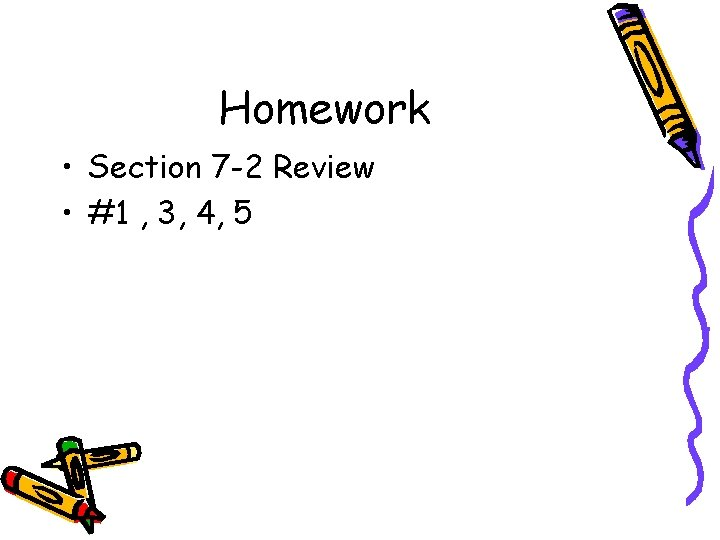Homework • Section 7 -2 Review • #1 , 3, 4, 5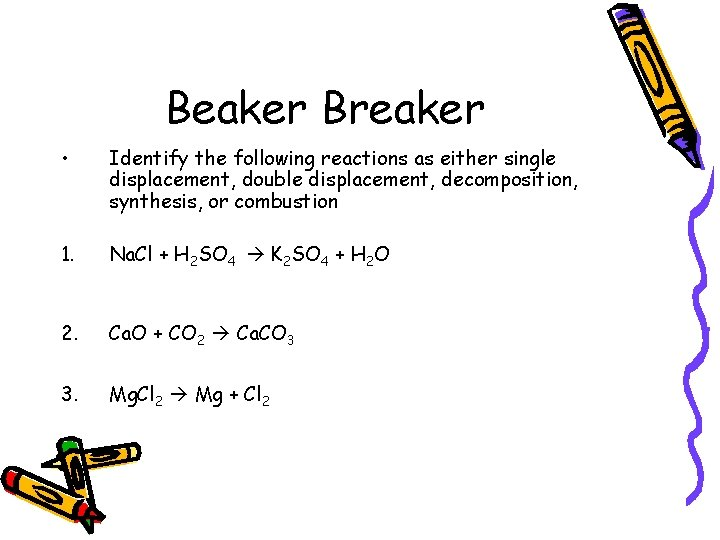Beaker Breaker • Identify the following reactions as either single displacement, double displacement, decomposition, synthesis, or combustion 1. Na. Cl + H 2 SO 4 K 2 SO 4 + H 2 O 2. Ca. O + CO 2 Ca. CO 3 3. Mg. Cl 2 Mg + Cl 27 -3 Continued Exothermic and Endothermic Reactions • During a chemical reaction, energy is either ____ or ______ • A chemical reaction that_____ energy to its surroundings is called an_______ reaction • Ex: Freezing • A chemical reaction that_____ energy from its surroundings is called an______ • Ex: MeltingExothermic Reactions • In exothermic reactions, the energy released as the products form is _____ than the energy required to break the bonds in the reactants • Example – Combustion • C 3 H 8 + 5 O 2 3 CO 2 + 4 H 2 O + 2220 k. J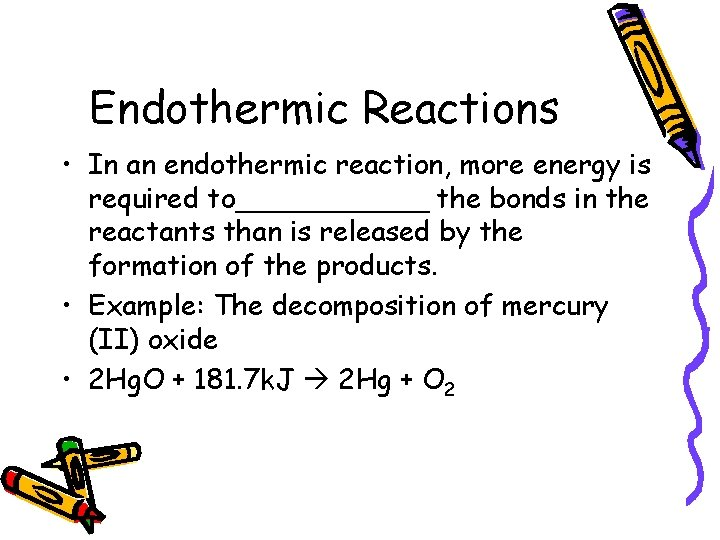Endothermic Reactions • In an endothermic reaction, more energy is required to______ the bonds in the reactants than is released by the formation of the products. • Example: The decomposition of mercury (II) oxide • 2 Hg. O + 181. 7 k. J 2 Hg + O 2Conservation of Energy • The law of__________ states in exothermic and endothermic reactions the total amount of energy before and after the reaction is the same.Homework • 7 -3 Section Review page 209 • Numbers 1, 2, 3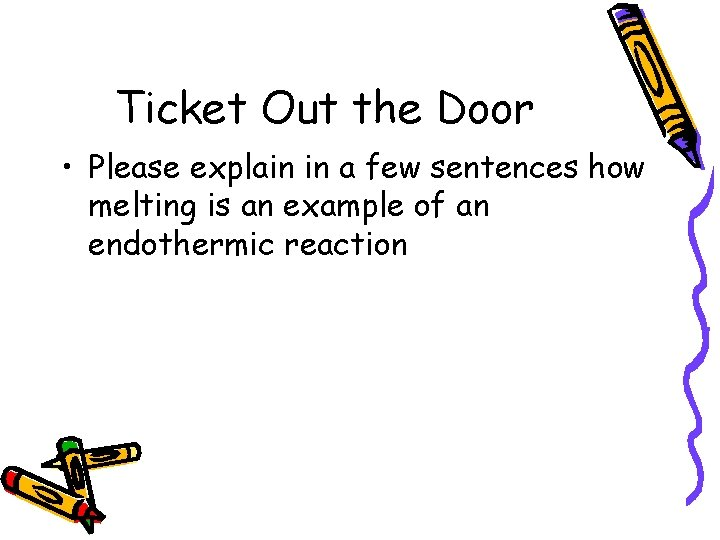Ticket Out the Door • Please explain in a few sentences how melting is an example of an endothermic reaction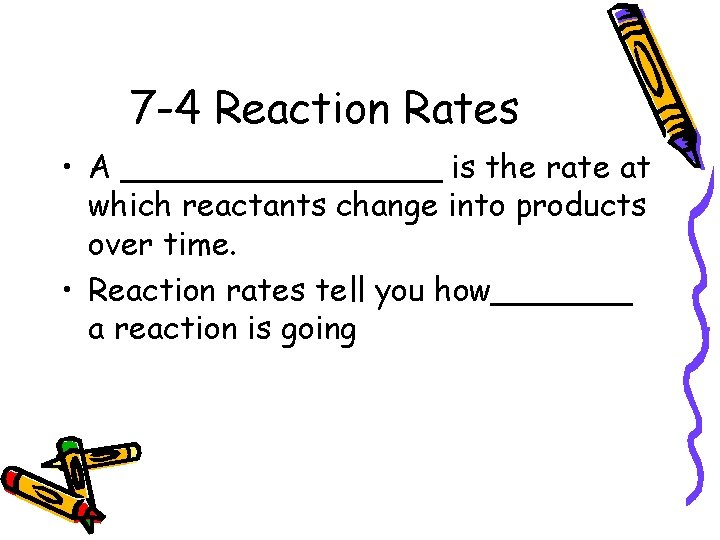7 -4 Reaction Rates • A ________ is the rate at which reactants change into products over time. • Reaction rates tell you how_______ a reaction is going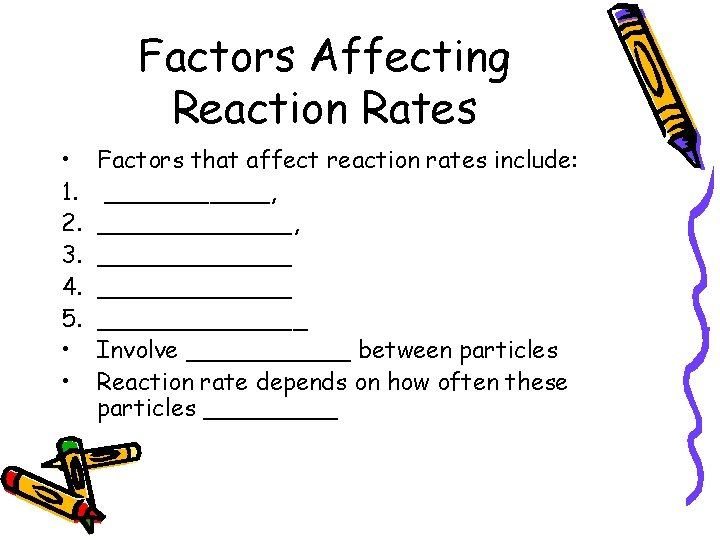Factors Affecting Reaction Rates • 1. 2. 3. 4. 5. • • Factors that affect reaction rates include: ___________, _____________ Involve ______ between particles Reaction rate depends on how often these particles _____Temperature • An_____ in temperature will _____the reaction rate • Ex. Frying an egg • ____ the temp will ____ the reaction rate. • Ex: refrigerating milk to prevent spoiling • Increasing the ____, increases the number of collisions between the particles, then _____ increasesSurface Area • The ____ the particle size of a given mass, the _______ is its surface area. • An increase in _____ increases the _____ of reactants to one another. • The _____this exposure, the more collisions there are that involve reacting particles. • ____ the surface area of a reactant tends to_____ the reaction rate.Beaker Breaker • • • A_____rate is the rate at which reactants change into products over time List two factors that affect reaction rates: An _____ in temperature will_____ the reaction rateStirring • You can ______ the exposure of reactants to each other by _____ • Ex: washing machine • Stirring _______ the reaction. • ______ between the particles of the reactants are more likely to happenConcentration • _______ refers to the number of particles in a given volume • Ex: concentrated dyes • The reaction rate is ______ • For gases, concentration changes with________ of a gaseous reactant, the _____ is its concentration, and the_____ the reaction rate.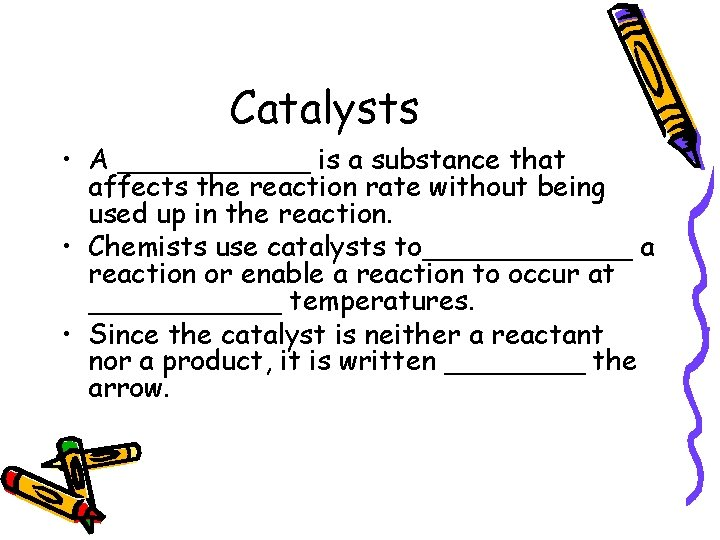Catalysts • A ______ is a substance that affects the reaction rate without being used up in the reaction. • Chemists use catalysts to______ a reaction or enable a reaction to occur at ______ temperatures. • Since the catalyst is neither a reactant nor a product, it is written ____ the arrow.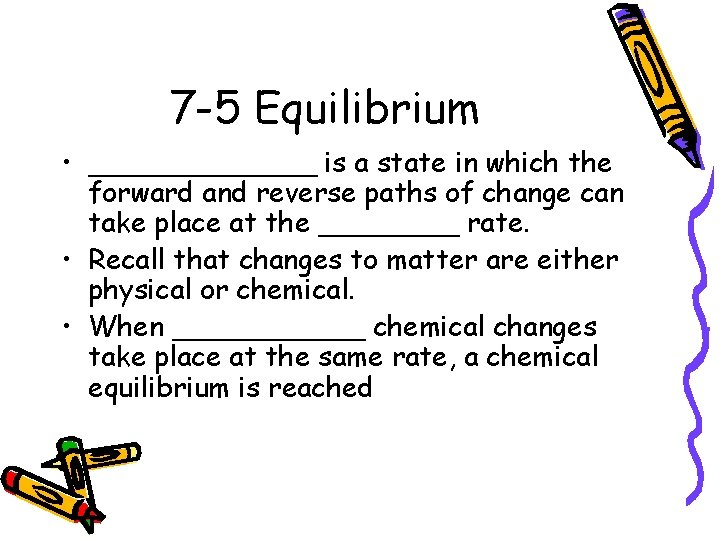7 -5 Equilibrium • _______ is a state in which the forward and reverse paths of change can take place at the ____ rate. • Recall that changes to matter are either physical or chemical. • When ______ chemical changes take place at the same rate, a chemical equilibrium is reachedPhysical Equilibrium • When a _____ change does not go to completion, a physical equilibrium is established between the forward and reverse changes • Ex: H 2 O (l) H 2 O(g) _____and______are both physical changes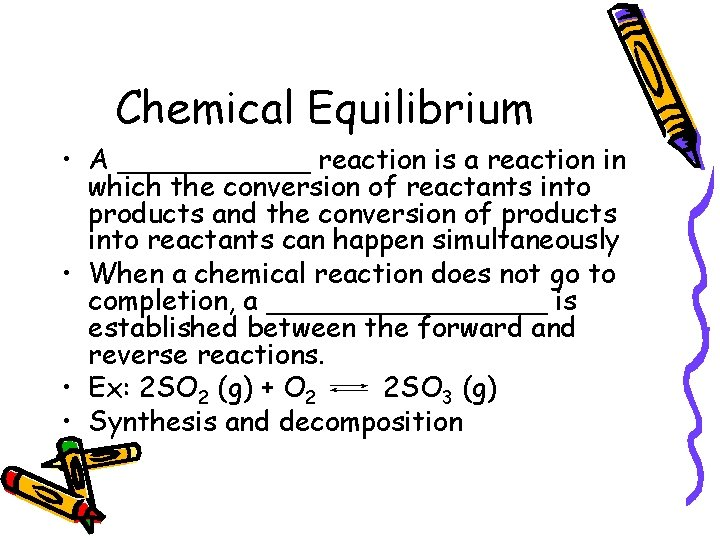Chemical Equilibrium • A ______ reaction is a reaction in which the conversion of reactants into products and the conversion of products into reactants can happen simultaneously • When a chemical reaction does not go to completion, a ________ is established between the forward and reverse reactions. • Ex: 2 SO 2 (g) + O 2 2 SO 3 (g) • Synthesis and decomposition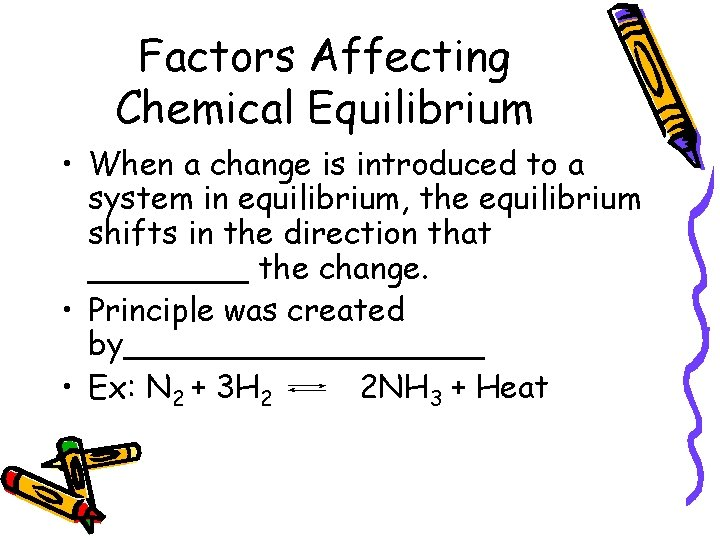Factors Affecting Chemical Equilibrium • When a change is introduced to a system in equilibrium, the equilibrium shifts in the direction that ____ the change. • Principle was created by_________ • Ex: N 2 + 3 H 2 2 NH 3 + HeatTemperature • N 2 + 3 H 2 2 NH 3 + Heat • Synthesis of ammonia, heat is written as a product • Forward reaction is ______ • Reverse is decomposition and is ______ • Adding heat would then favor the_______reaction, which is endothermic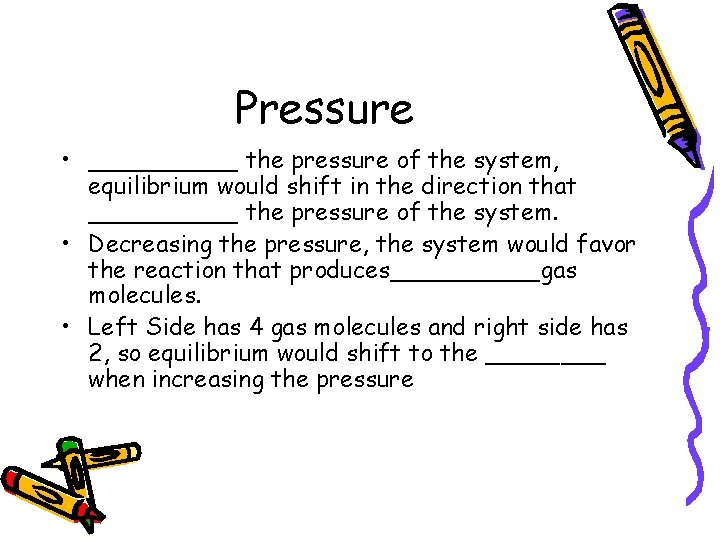Pressure • _____ the pressure of the system, equilibrium would shift in the direction that _____ the pressure of the system. • Decreasing the pressure, the system would favor the reaction that produces_____gas molecules. • Left Side has 4 gas molecules and right side has 2, so equilibrium would shift to the ____ when increasing the pressure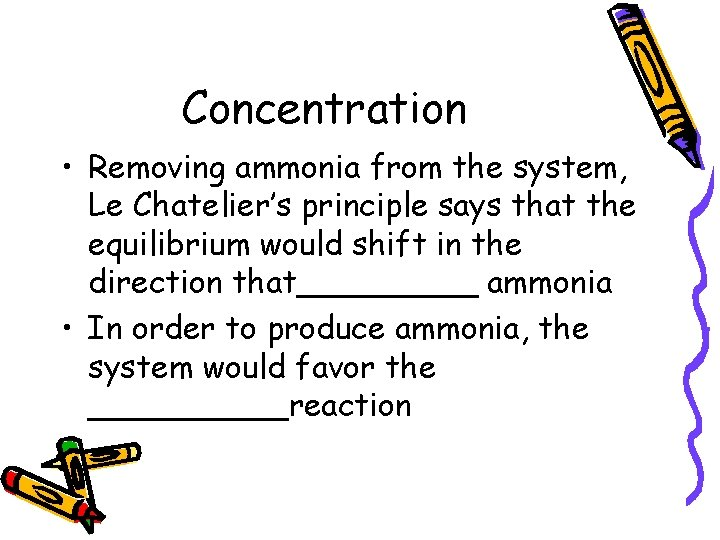Concentration • Removing ammonia from the system, Le Chatelier’s principle says that the equilibrium would shift in the direction that_____ ammonia • In order to produce ammonia, the system would favor the _____reaction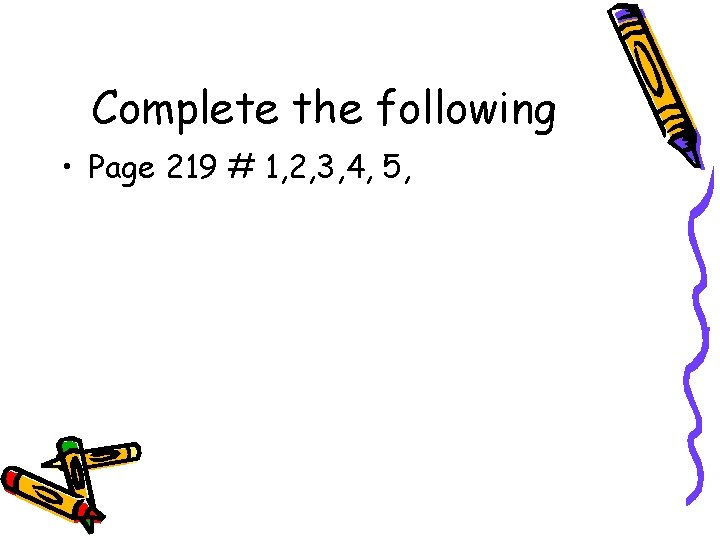Complete the following • Page 219 # 1, 2, 3, 4, 5,